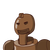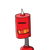# Find the cost of 6 kg sugar if the cost of 4 kg sugar is Rs. 160​

Find the cost of 6 kg sugar if the cost of 4 kg sugar is Rs. 160​

### 2 thoughts on “Find the cost of 6 kg sugar if the cost of 4 kg sugar is Rs. 160​”

1.4 kg = 160

1kg = 160/4

1 kg = 40

6 kg = 40 × 6

6 kg = ₹240

2.hi free fire keltha hai kya tu